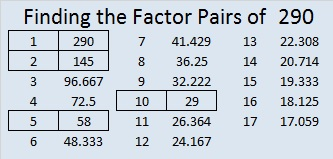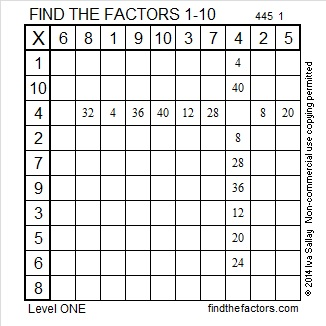# 290 and Level 1

• 290 is a composite number.
• Prime factorization: 290 = 2 x 5 x 29
• The exponents in the prime factorization are 1, 1, and 1. Adding one to each and multiplying we get (1 + 1)(1 + 1)(1 + 1) = 2 x 2 x 2 = 8. Therefore 290 has 8 factors.
• Factors of 290: 1, 2, 5, 10, 29, 58, 145, 290
• Factor pairs: 1 x 290, 2 x 145, 5 x 58, or 10 x 29
• 290 has no square factors that allow its square root to be simplified. √290 ≈ 17.029Print the puzzles or type the factors on this excel file: 10 Factors 2014-11-10This site uses Akismet to reduce spam. Learn how your comment data is processed.